Related Articles

# Volume and Capacity – Mensuration | Class 8 Maths

Mensuration is the branch of mathematics that talks about the length, volume, and area of different geometrical objects. The shape may be in 2-D or 3-D. We find the volume of 3-D objects and area of 2-D objects.

### What is Volume?

Volume is the measurement of total space occupied by a given solid. Volume is defined in 3-D because to have the volume the object must have three variables i.e. length, breadth or thickness or width, and height.

The difference between the total amount of space left inside the hollow body and the space occupied by the body is the volume of the figure.

Now, let us take the example of a 3d shape called cuboid, it is a solid figure bounded by six rectangles and thus has six rectangular faces. The dimensions of the cuboid are as follows: length l, breadth b, and height h.height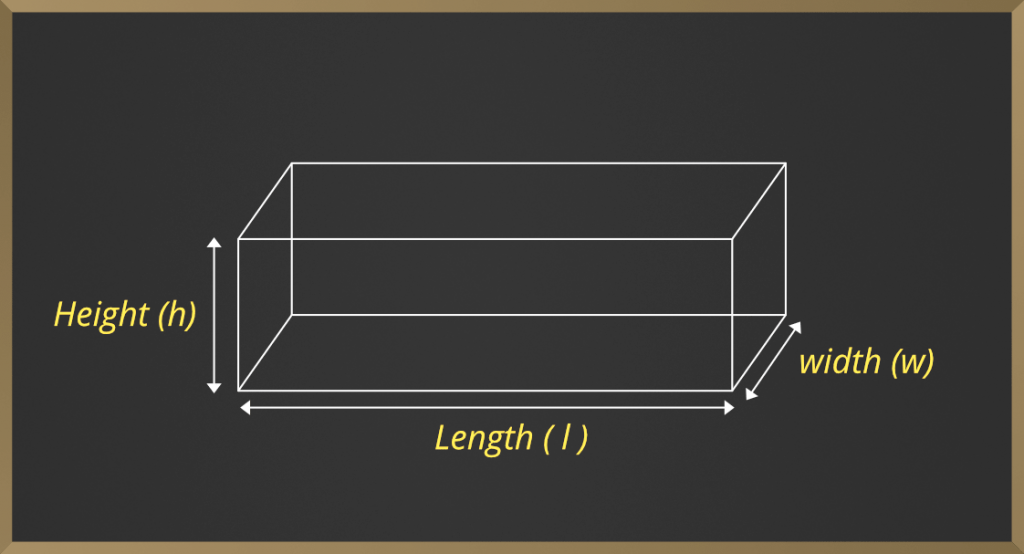Volume of the cuboid is given as:

Volume = length * height * width

Volume is for both solid and hollow objects. E.g. Cube, Cuboid, Cone, Cylinder, etc.

### What is capacity?

When the hollow object is filled with liquid or air and it takes the shape of that object or container. The total volume of the water and air which is filled inside the container is called the capacity of the container.

Note: Capacity is calculated only for hollow object.

Example: Cone, Cylinder, Hollow hemisphere, etc.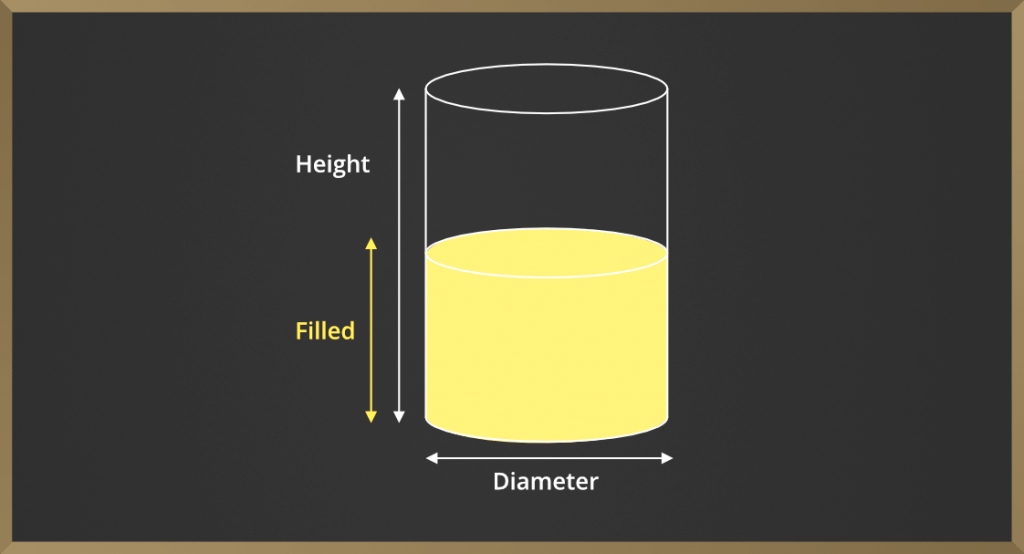Example: Find the volume and capacity of cylinder whose radius is 14 cm and height is 21cm.

Solution: We have given, r = 14cm, h = 21cm;

Volume of cylinder = πr*r*r*h.

V = 22/7 * 14 * 14 * 21

V = 12,936cm3.

and capacity is = 12,936/1000 litre     …….as (1000cm3= 1litre)

= 12.936 litre.

Note: The unit of capacity is litres(l) and millilitres(ml).

## Mensuration of Some Enclosed Figures

### Cube

Surface area of cube is defined as the area of every face of the cube. For e.g. as we know that every side of cube is equal. So area of one face is  length*length or width*width or height*height  out of these three we pick one according to question.

So total surface area of cube is 6 * length * length. Because cube has six faces and each face has an area of length*length.

Now the volume of cube is its hollow portion and can be written as length * length * length or width * width * width or height * height * height. And its unit will be cubic.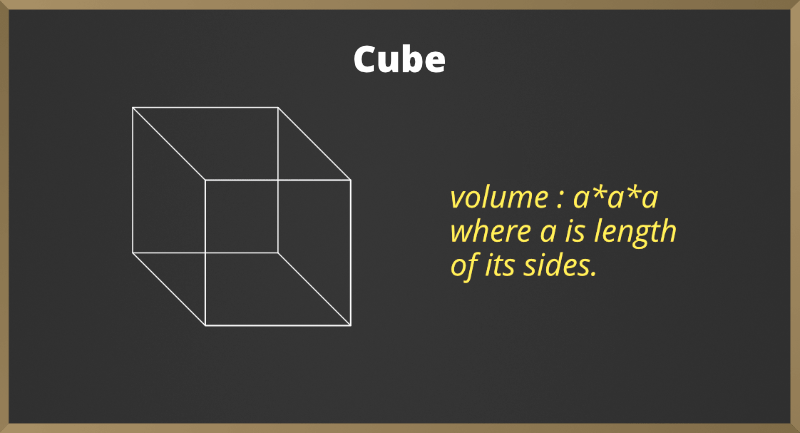Example: Find the width of the cube whose volume is 625cm3.

Solution:

We know that volume of the cube is a * a * a =a3 where a  can be the length, width, height.

So, a3 = 125 then

a = 5cm ( 5 is cube root of 125)

So width is 5 cm.

### Cuboid

Surface area of cuboid is defined as the surface area of cube but the difference here is all the sides are not equal and in cuboid length width height are different. So its surface area will be the area of each face and add all the areas which we will get total surface area.

So total surface area of cuboid is length * width + width * height + height * length + length * width + width * height + height * length i.e. the addition of areas of every six face

So total surface area is

2 * (length * width + width * height + height * length)

The volume of cuboid is the hollow portion inside the cuboid and it is

Volume = length * width * height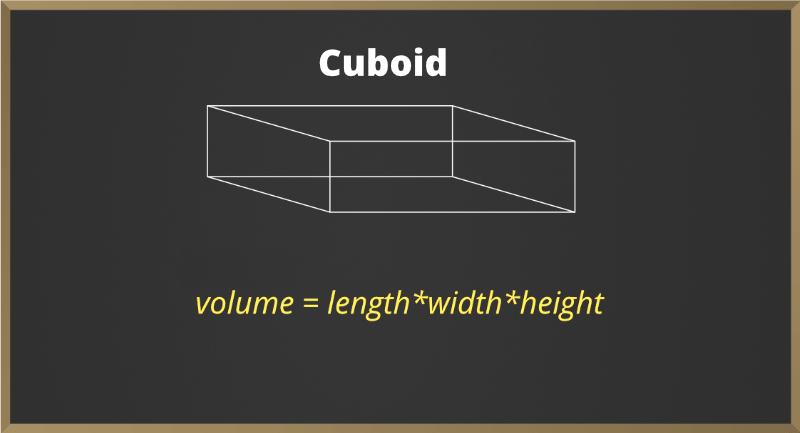Example: Find the height of cuboid volume is 625cm3 and its base area is 25 cm2.

Solution:

Base area of cube means width * length this forms base in cube

As we know volume of cube is

=> l * b * h = 625

=> h = 625 / b * l

=> h = 625 / 25

=> h = 25cm

So the height is 25 cm

### Cylinder

Here we are talking about right circular cylinder for e.g. round pillar, tube lights, water pipes, etc. Volume of cylinder is the hollow portion inside the cylinder.

Volume of Cylinder = 22/7 * r * r * h

Here,

r = radius of cylinder and h = height of cylinder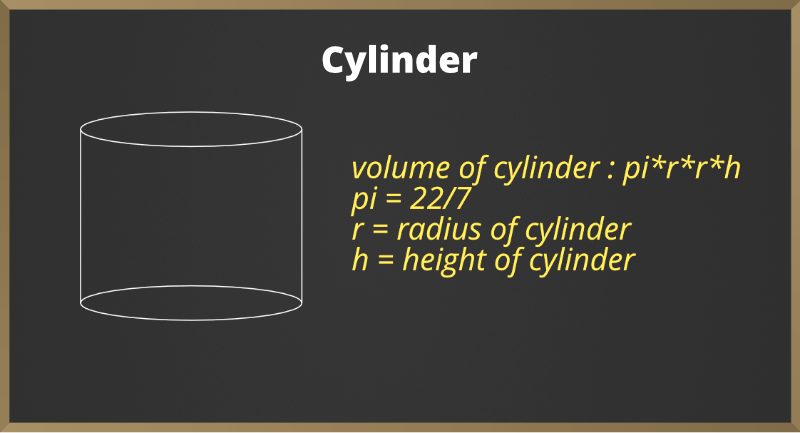Example: A rectangular sheet of paper having length 11cm and width 4cm  cm is being rolled to form a cylinder of height 4 cm. What is the volume of the cylinder?

Solution:

Let the cylinder if radius = r and its height = h

Perimeter of  base of cylinder = 2 * pi * r = 11cm

=> 2 * 22/7 * r = 11cm

=> r = 7/4 cm

Volume = 22/7 * r* r * h

=> 22/7 * 7/4 * 7/4 * 4

=> 38.5cm3

Hence, the volume of cylinder is 38.5cm3

### Cone

Volume of cone is the hollow portion inside cone.

Volume = 1/3 * 22/7 * r * r * h

Here,

h = height of cone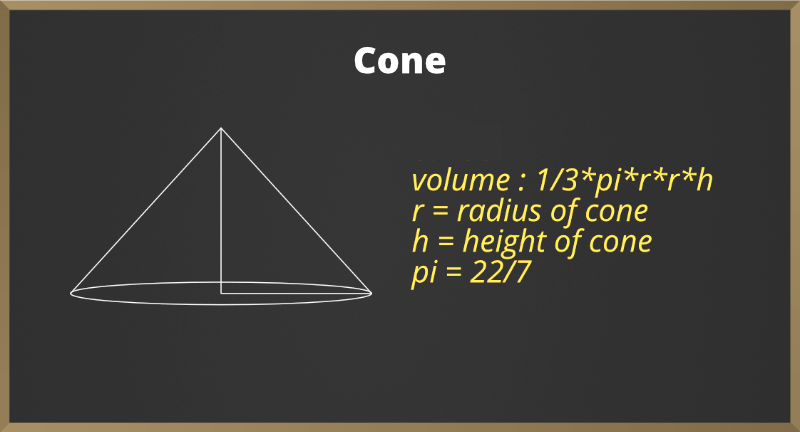### Sample Problems on Volume and Capacity

Problem 1: Find the capacity, in litres, of cubic tank with length, width, height is given as 1 m, 1m, and 1m respectively.

Solution:

First we convert between volume and capacity in cm3 and ml so we need to find volumes in cm3

The volume is 1m * 1m * 1m = 1m as it is cube so we used cube formula

As 1m = 100cm so volume in cm3 is 100cm * 100cm * 100cm = 1000000cm3

As 1cm3 = 1ml So the capacity of the tank in ml is 1000000ml.

and finally capacity of tank in litre is 1000000/1000=1000litres.

As 1000ml is 1 litre. So the capacity of tank is 1000litres.

Problem 2: A tank measures 2m, 1m, 2m. Find the capacity of tank.

Solution:

As we know that 1m = 100cm.

As it is cuboid figure because its length is different.

Volume of cuboid = length * width * height = 2m * 1m * 2m = 4m3.

Or 200cm * 100cm * 200cm = 4000000cm3.

We know that, 1cm3 = 1ml so 4000000cm3 = 4000000ml

As 1000ml = 1litre,  then 4000000ml is 4000000/1000 = 4000 litres is capacity of tank.

Problem 3: A rectangular sheet of paper is being rolled and converted to cylinder of radius 10cm  what is the volume of cylinder? Width of rectangle is 7cm.

Solution:

Width of the rectangle becomes the height of the cylinder and the radius of the cylinder is given as 10 cm.

So the value of cylinder is V = 22/7 * r * r * h

V = 22/7 * 10 * 10 * 7

v = 22 * 10 * 10

v = 2200cm3.

Problem 4: What is the volume of the cone if the radius is 9cm and height is 14cm?

Solution:

The volume of cone = 1/3 * pi * r * r * h

Hence, volume is 1/3 * 22/7 * 9 * 9 * 14

1 * 22 * 3 * 9 * 2 = 1,188cm3.

Whether you're preparing for your first job interview or aiming to upskill in this ever-evolving tech landscape, GeeksforGeeks Courses are your key to success. We provide top-quality content at affordable prices, all geared towards accelerating your growth in a time-bound manner. Join the millions we've already empowered, and we're here to do the same for you. Don't miss out - check it out now!

Previous
Next